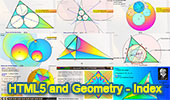# HTML5 and Dynamic GeometryLangley's Problem: HTML5 animation' Adventitious angles. 20° isosceles triangle with animation. Dynamic Geometry 1481. Five Tangential or Circumscribed Quadrilaterals, Pitot Theorem, Congruence, Step-by-step Illustration. GeoGebra, iPad. Dynamic Geometry 1480. Japanese Theorem for Cyclic Polygon, Sangaku, Triangulation, Non-intersecting Diagonals, Sum of Inradii, Invariant, Step-by-step Illustration. GeoGebra, iPad. Dynamic Geometry 1479. Triangle, Circumcircle, Angle Bisector, Perpendicular Bisector, Chord, Concyclic Points, Parallel Lines,, Step-by-step Illustration. GeoGebra, iPad. Dynamic Geometry 1478. Cyclocevian, Reuschle-Terquem Theorem, Concurrent Cevians, Triangle, Circumcircle, Secant line, Step-by-step Illustration. GeoGebra, iPad. Dynamic Geometry 1477. Miquel's Pentagram Theorem, Pentagon, Triangle, Circumcircles, Concyclic Points, Step-by-step Illustration. GeoGebra, iPad. Dynamic Geometry 1476. Droz-Farny Line Theorem, Triangle, Orthocenter, Perpendicular, Collinear Midpoints, Step-by-step Illustration. GeoGebra, iPad. Dynamic Geometry 1475. Clifford Intersecting Circles Theorem, Step-by-step Illustration, GeoGebra, iPad. Dynamic Geometry 1474. Butterfly Theorem, Circle, Chords, Midpoints, Step-by-step Illustration. Dynamic Geometry 1473. Kosnita's Theorem, Triangle, Four Circumcenters, Concurrent Line, Step-by-step Illustration. Dynamic Geometry 1472. Cyclic Quadrilateral, Perpendicular Diagonals, Rectangle, Step-by-step Illustration. Dynamic Geometry 1471. Equilateral Triangle, Inside/Outside Point, Incenters, Tangency Points, Concurrent Lines, Step-by-step Illustration. Dynamic Geometry 1470. Tangential Quadrilateral, Incircles, Tangent, Parallel, Rhombus, Step-by-step Illustration. Dynamic Geometry 1469. Triangle, Circumradius, Inradius, Midpoints, Arcs, Sum of Distances, Step-by-step Illustration. Dynamic Geometry 1468. Steiner's Theorem, Triangle, Circumradius, Inradius, Sum of Exradii, Step-by-step Illustration. Geometry Problem 1466. Tangential Quadrilateral, Newton Line, Incenter, Midpoint, Diagonal, Collinear Points. Step-by-step Illustration using GeoGebra. Geometry Problem 1465. Tangential Quadrilateral, Incenter, Inscribed Circle, Equal Sum of Areas. Step-by-step Illustration using GeoGebra. Geometry Problem 1464. Quadrilateral, Interior Point, Midpoint of Sides, Equal Sum of Areas. Step-by-step Illustration using GeoGebra. Geometry Problem 1463. Parallelogram, Interior Point, Opposite Triangles with Equal Sum of Areas. Step-by-step Illustration using GeoGebra. Geometry Problem 1462. Newton-Line, Newton-Gauss Line, Complete Quadrilateral, Midpoints of Sides and Diagonals, Collinear Points. Dynamic Geometry 1460. Newton-Gauss Line, Complete Quadrilateral, Midpoints of Diagonals, Collinear Points, Step-by-step Illustration using GeoGebra. Geometry Problem 1459. Two Triangles, Orthocenter, Midpoint, Perpendicular, Step-by-step Illustration using GeoGebra. Geometry Problem 1458. Triangle, Incircles, Excircle, Area, Step-by-step Illustration using GeoGebra. Geometry Problem 1457. Altitudes, Circles, Similarity, Product of the Inradii Lengths, iPad. Step-by-step illustration using GeoGebra. Geometry Problem 1456. Altitudes, Orthic Triangle, Circumcircle, Parallel lines, Similarity, Area, iPad. Step-by-step illustration using GeoGebra. Geometry Problem 1455. Nagel Point, Excircles, Incircle, Congruent Segments, iPad. Step-by-step illustration using GeoGebra. Geometry Problem 1454. Intersecting Circles, Perpendicular Lines, Cyclic Quadrilateral, Collinear Points, iPad. Step-by-step illustration using GeoGebra. Dynamic Geometry 1452. Japanese Theorem, Sangaku, Cyclic Quadrilateral, Incenter, Rectangle, Inradius. Step-by-step illustration using GeoGebra. Dynamic Geometry 1451. Orthopole of a Line. Step-by-step illustration using GeoGebra. Dynamic Geometry 1450. Ortholine, Steiner Line, Complete Quadrilateral, Collineal Orthocenters. Step-by-step illustration using GeoGebra. Dynamic Geometry 1449. Salmon Line. Step-by-step illustration using GeoGebra. Dynamic Geometry 1448. Simson Line. Step-by-step illustration using GeoGebra. Dynamic Geometry Problem 1447. Outer Vecten Point. Step-by-step illustration using GeoGebra. Dynamic Geometry Problem 1446. Lemoine Line, triangle, circumcircle, tangent, collinear points. Step-by-step animation using GeoGebra. Dynamic Geometry Problem 1445. Van Aubel's theorem, Quadrilateral and Four Squares, Centers. Step-by-step animation using GeoGebra. Dynamic Geometry Problem 1444. The Asymmetric Propeller Theorem, Equilateral Triangles, Midpoints. Using GeoGebra. First Ajima-Malfatti Point: HTML5 animation. Step-by-step animation. Second Ajima-Malfatti Point: HTML5 animation. Interactive illustration. Apollonius' Problem for Three Circles: HTML5 animation. Interactive illustration. Equal Incircles Theorem: HTML5 animation. Step-by-Step Illustration. Geometric Art using iPad Apps Eyeball Theorem: HTML5 version. Animated Angle to Geometry Study. Miquel's Pentagram Theorem: HTML5 version.  Interactive proof with animation and key theorems. Nine-Point Center, Nine-Point Circle, Euler Line: HTML5 Animation for iPad.Circumcenter, Centroid, Orthocenter. Euler and his beautiful and extraordinary formula that links the 5 fundamental constants in Mathematics, namely, e, i, Pi , 1 and 0, together! HTML5 Animation for iPad and Nexus Nagel Point Theorem. Proof. Triangle and excircles. Animation.  Adobe Flash. Seven Circles Theorem. Animation. Adobe Flash. Ceva's Theorem. Concurrency. Interactive proof with animation. Key concept: Menelaus Theorem. Menelaus' Theorem. Interactive proof with animation and key concepts.. Simson Line. Animation, Proof. Adobe Flash.Home | Sitemap | Search | Geometry | Dynamic GeometryEmail | by Antonio Gutierrez
Last updated May 24, 2020